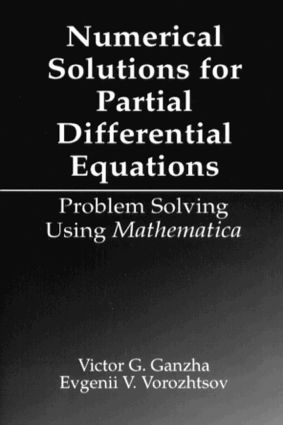# Numerical Solutions for Partial Differential Equations

## Problem Solving Using Mathematica, 1st Edition

CRC Press

347 pages

##### Purchasing Options:\$ = USD
Hardback: 9780849373794
pub: 1996-07-12
SAVE ~\$23.00
\$115.00
\$92.00
x
eBook (VitalSource) : 9780203745311
pub: 2017-11-22
from \$57.50

FREE Standard Shipping!

### Description

Partial differential equations (PDEs) play an important role in the natural sciences and technology, because they describe the way systems (natural and other) behave. The inherent suitability of PDEs to characterizing the nature, motion, and evolution of systems, has led to their wide-ranging use in numerical models that are developed in order to analyze systems that are not otherwise easily studied. Numerical Solutions for Partial Differential Equations contains all the details necessary for the reader to understand the principles and applications of advanced numerical methods for solving PDEs. In addition, it shows how the modern computer system algebra Mathematica® can be used for the analytic investigation of such numerical properties as stability, approximation, and dispersion.

### Reviews

:"…A completely new edition designed for an era of calculators and handheld computers."

@:- James A. Cargal, Troy State University, Montgomery, Alabama, in The Journal of Undergraduate Mathematics and Its Applications, 1996

@

### Table of Contents

Introduction to Mathematica

General Information about Mathematica

Symbolic Computations with Mathematica

Numerical Computations with Mathematica

Finite Difference Methods for Hyperbolic PDEs

Construction of Difference Schemes for the Advection Equation

The Notion of Approximation

Fourier Stability Analysis

Elementary Second-Order Schemes

Algorithm for Automatic Determination of Approximation Order of Scalar Difference Schemes

Monotonicity Property of Difference Schemes

TVD Schemes

The Construction of Difference Schemes for Systems of PDEs

Implicit Difference Schemes

Von Neumann Stability Analysis in the Case of Systems of Difference Equations

Difference Initial- and Boundary-Value Problems

Construction of Difference Schemes for Multidimensional Hyperbolic Problems

Determination of Planar Stability Regions

Curvilinear Spatial Grids

Answers to the Exercises

Finite Difference Methods for Parabolic PDEs

Basic Types of Boundary Conditions for Parabolic PDEs

Simple Schemes for the One-Dimensional Heat Equation

Difference Schemes for Advection-Diffusion Equation

Runge-Kutta Methods

Finite Volume Method

The Adi Method

Approximate Factorization Scheme

Dispersion

Answers to the Exercises

Numerical Methods for Elliptic PDEs

Boundary-Value Problems for Elliptic PDEs

A Simple Elliptic Solver

Pseudo-Unsteady Methods

The Finite Element Method

Numerical Grid Generation

Local Approximation Study of Finite Volume Operators on Arbitrary Grids

Local Approximation Study of Difference Schemes on Logically Rectangular Grids

Answers to the Exercises

Appendix Glossary of Programs

Index

Each Chapter also includes a list of references.

### Subject Categories

##### BISAC Subject Codes/Headings:
MAT000000
MATHEMATICS / General
MAT003000
MATHEMATICS / Applied
MAT007000
MATHEMATICS / Differential Equations
MAT021000
MATHEMATICS / Number Systems